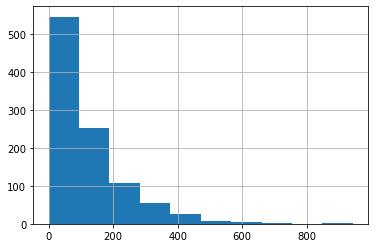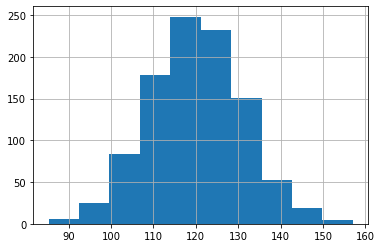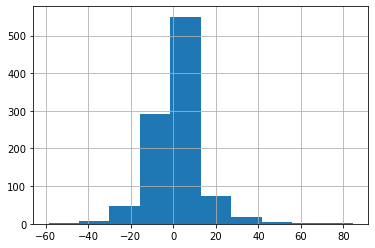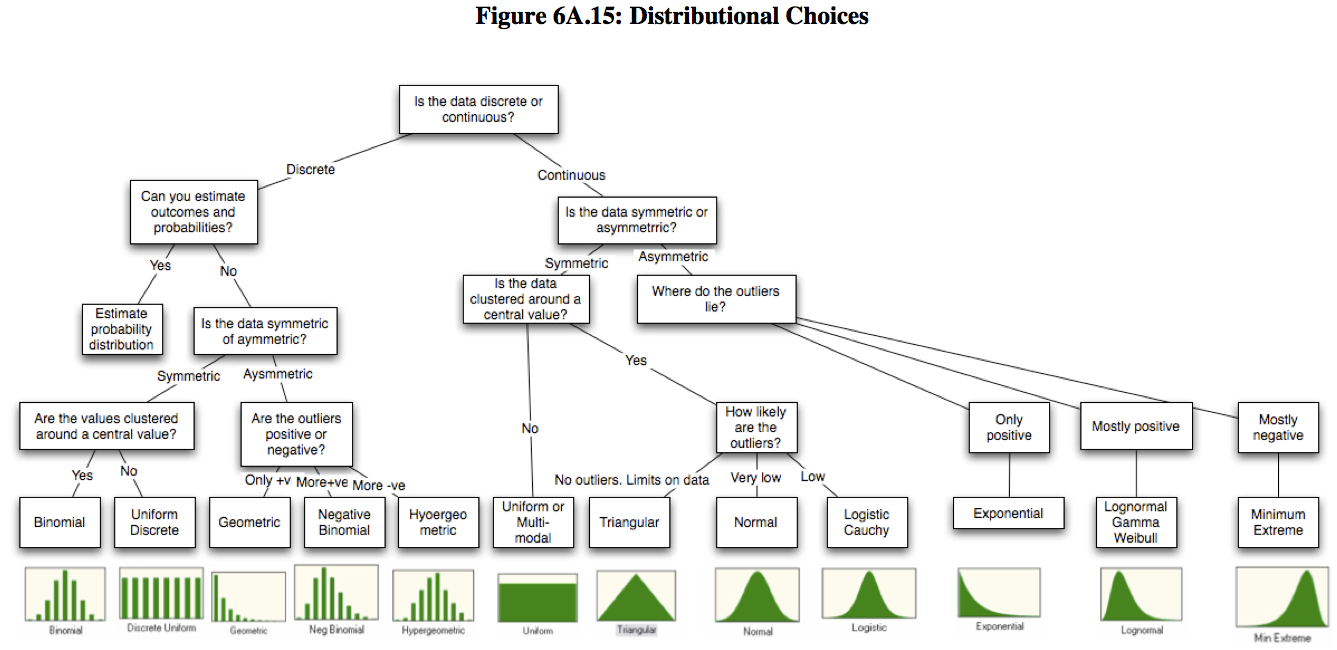## Why create statistical distributions?

As a simulation engineer, one of my responsibilities is to create statistical experiments to simulate various scenarios and understand the outcomes. These outcomes have probabilities associated with them which help the decision makers to identify key risks, uncertainties and make informed decisions to leverage risk. For example, if the company wants to invest a million dollars in a capital project, there are many uncertainties involved such as labor costs, scheduling uncertainty, project delays, technical risks etc. A statistical simulation experiment, i.e Monte Carlo simulation, will assign probabilities with these risks and simulate thousands of outcomes to compute the probability of success. The company can then decide if it wants to invest the \$1M or what decisions it can take proactively to de-risk the uncertainties.

There are many statistical packages and Excel add-ons available to create such experiments but none that I know of in Power BI. In Power BI, only uniform distribution and normal distributions are available natively in DAX. In this blog post I share the DAX codes I use to create different distibutions.

If you are new to Monte Carlo / Discrete Event simulation, these distributions may not make sense. In a future blog post I will show when to use these distributions and how to create sophisticated simulations in Power BI. The beauty of creating it in Power BI vs Excel is that the stakeholders can interact with the simulation parameters and understand the probable outcomes much more clearly. The DAX codes given here can be used to create calculated tables for simulations.

If you are interested in this topic, please also take a look at my other blog on generating random numbers in Power BI

### Uniform Distribution

Uniform distribution to create values between 100 & 400.

DAX:

`Uniform = RANDBETWEEN(100,400)`### Normal Distribution

Normal distribution with mean 10, std dev of 1.

DAX:

```````Normal = NORM.INV(RAND(),10,1)`
```````### LogNormal Distribution

LogNormal distribution with mean = 80, variance = 225

DAX:

``````LogNormal =

VAR _m = 80 // mean
VAR _v = 225 // variance (not std deviation)
VAR _phi =
SQRT ( _v + _m ^ 2 )
VAR _mu =
LN ( ( _m ^ 2 ) / _phi ) // scaled mean
VAR _sigma =
SQRT ( LN ( ( _phi ^ 2 ) / _m ^ 2 ) )
RETURN

// scaled s.d

EXP ( NORM.INV ( RAND (), _mu, _sigma ) )``````### Beta PERT

Beta PERT with: Min likely value = 100 Med likely value = 300 Max likely value = 800

DAX:

``````Beta Pert =

VAR _a = 100
VAR _b = 300
VAR _c = 800
VAR _alpha = ( 4 * _b + _c - 5 * _a ) / ( _c - _a )
VAR _beta = ( 5 * _c - _a - 4 * _b ) / ( _c - _a )
RETURN
BETA.INV ( RAND (), _alpha, _beta, _a, _c )``````### Discrete

Discrete with:

Probability of Choice 1 = 50% , Probability of Choice 2 = 30% , Probability of Choice 3 = 20%

DAX:

``````Discrete =
IF (
'Table'[random_chocie] <= 0.5,
"Choice 1",
IF (
( 'Table'[random_chocie] > 0.5
&& 'Table'[random_chocie] < 0.8 ),
"Choice 2",
IF ( ( 'Table'[random_chocie] > 0.8 ), "Choice 3" )
)
)``````### Exponential Distribution

Exponential with lambda = 120

DAX:

``````Exponential =

VAR lambda = 120
RETURN
// Exponential distribution with a mean of lambda
-1 * lambda
* LN ( 1 - RAND () )``````### Poisson Distribution

Poisson distribution with mean = 120

DAX: Poisson =

``````VAR lambda = 120
RETURN
// Poisson dist with a mean of lambda
NORM.INV (
RAND (),
lambda,
SQRT ( lambda )
)``````### Truncated Normal Distribution

Truncated normal distribution with: lower limit = 1 higher limit = 4 mean = 3 s.d = 0.9

DAX: truncatednormal =

``````VAR _a = 1
VAR _b = 4
VAR _mu = 3
VAR _sigma = 0.9
RETURN
NORM.INV (
NORM.DIST ( _a, _mu, _sigma, TRUE )
+ RAND ()
* (
NORM.DIST ( _b, _mu, _sigma, TRUE )
- NORM.DIST ( _a, _mu, _sigma, TRUE )
),
_mu,
_sigma
)``````### Weibull Distribution

Weibull distribution with: shape parameter, alpha = 2 scale parameter, beta = 10

DAX

``````Weibull =

VAR _alpha = 2 // shape parameter
VAR _beta = 10 // scale parameter
RETURN

_beta
* ( - LN ( 1 - RAND () ) ) ^ ( 1 / _alpha )``````### Logistic Distribution

Logistic distribution with: location parameter, a = 2 scale parameter, k = 10

DAX

``````Logistic =

VAR _a = 2  // Location Parameter
VAR _k = 10 // Scale Parameter

RETURN
_a
+ _k
* LN ( ( RAND () / ( 1 - RAND () ) ) )``````### Laplace Distribution

Laplace distribution with: mean = 10 std dev = 2

DAX

``````Laplace =

VAR _rand = 'Table'[random_chocie]
VAR _mu = 10
VAR _sigma = 2
RETURN
IF (
_rand <= 0.5,
_mu
+ LN ( 2 * _rand ) / _sigma,
10
- LN ( 2 - 2 * _rand ) / _sigma
)``````### Gumbel Distribution / Extreme Value Distribution

Gumbel/EV Distribution with: mean = 10 std dev = 2

DAX

``````Gumbel =

VAR _mu = 10
VAR _sigma = 2
RETURN

_mu
- ( _sigma * LN ( - LN ( RAND () ) ) )``````You may find below visual helpful in determining which distribution to use: